• n×nn\times n矩阵的是另一个n×nn\times n矩阵，AA的写成A−1A^{-1}，它的基本性质是：如果乘AA后再乘以A−1A^{-1}，那么将回到开始状态： 如果b=Ax,那么A−1b=x \text{如果}b=Ax,\text{那么}A^{-1}b=x 因此A...

n×n $n\times n$矩阵的逆是另一个 n×n $n\times n$矩阵， A $A$的逆写成A1$A^{-1}$，它的基本性质是：如果乘 A $A$后再乘以A1$A^{-1}$，那么将回到开始状态：

b=Ax,A1b=x
因此 A1Ax=x $A^{-1}Ax=x$。矩阵 A1×A $A^{-1}\times A$得到单位矩阵，然而并非所有矩阵都存在逆，当 Ax=0 $Ax=0$ x0 $x\neq0$时矩阵不存在逆，也就是说当我们想找到矩阵 A1 $A^{-1}$使得 Ax=0 $Ax=0$变回 x $x$时，不存在这样的A1$A^{-1}$，因为我们不可能有矩阵乘以零向量 Ax $Ax$后得到非零向量 x $x$

当矩阵的逆A1$A^{-1}$存在时，我们的目标是定义，计算并使用它，还有需要知道什么样的矩阵不存在逆。

11、对于矩阵 A,B $A,B$，使得 BA=I,AB=I $BA=I,AB=I$，并且最多只有一个 B $B$，我们称它为A$A$的逆并用 A1 $A^{-1}$表示：

A1A=I,AA1=I(1)

1. 当且仅当消元过程得到 n $n$个主元(可以进行行交换)时矩阵的逆才存在，消元法在求解Ax=b$Ax=b$时没有显示的去找 A1 $A^{-1}$
2. 矩阵 A $A$不可能有两个逆，假设BA=I$BA=I$并且 AC=I $AC=I$，那么 B=C $B=C$。证明如下：
B(AC)=(BA)CBI=IC,(2)
这说明左逆 B $B$(从左边相乘)和右逆C$C$(从右边相乘)肯定相等。
3. 如果 A $A$可逆，那么Ax=b$Ax=b$的唯一解是 x=A1b $x=A^{-1}b$
A1Ax=b,x=A1Ax=A1b
4. (重要)假设有非零向量使得 Ax=0 $Ax=0$，那么 A $A$不存在逆。再说一遍：不存在这样的矩阵可以将0变回x$x$
5. 一个 2×2 $2\times 2$矩阵是可逆的，当且仅当 adbc $ad-bc$不等于零:
adbc $ad-bc$是矩阵 A $A$的行列式，如果它非零那么矩阵就是可逆的(这在之后的文章会讲到)。在matlab里面，判断是否可逆就是看是否能找到n$n$个主元，在行列式出现之前是用消元法产生这些主元的。
6. 对于对角矩阵，如果对角元素没有零，那么它存在逆：
A=d1dn,A1=1/d11/dnAA1=I
当涉及两个矩阵时，对于 A+B $A+B$的逆我们无法判断，它的和可能有也可能没有逆，但是他们的乘积是可逆的，这在矩阵乘法中是非常重要的公式。这和我们数的情况类似， (a+b)1 $(a+b)^{-1}$很难化简，而 1/ab $1/ab$可以分为 1/a×1/b $1/a\times 1/b$。但是对于矩阵乘法，顺序非常重要，如果 ABx=y $ABx=y$，那么 Bx=A1y,x=B1A1y $Bx=A^{-1}y,x=B^{-1}A^{-1}y$，逆的顺序正好相反。

12、乘积 AB $AB$的可逆矩阵是 B1A1 $B^{-1}A^{-1}$

(AB)1=B1A1(4)

证明：为了说明 B1A1 $B^{-1}A^{-1}$ AB $AB$的逆，我们将他们相乘并利用结合律去掉符号：
(AB)(B1A1)=ABB1A1=AIA1=AA1=I
(B1A1)(AB)=B1A1AB=BIB1=BB1=I
对于三个或更多的矩阵同样成立：
(ABC)1=C1B1A1
当用消元矩阵 E,F,G $E,F,G$ U $U$变回A$A$时，我们将会发现他们的顺序发生了变化。前向是 GFEA $GFEA$得到 U $U$，反向是L=E1F1G1$L=E^{-1}F^{-1}G^{-1}$，因为前向中 G $G$是最后一个，所以反向里G1$G^{-1}$是第一个。

计算 A−1 $A^{-1}$：Gauss-Jordan方法

考虑方程 AA1=I $AA^{-1}=I$，如果一次取 A1 $A^{-1}$的一列，那么 A1 $A^{-1}$的第一列乘以 A $A$得到单位矩阵的第一列：Ax1=e1$Ax_1=e_1$。同样的可得出 Ax2=e2,Ax3=e3 $Ax_2=e_2,Ax_3=e_3$，考虑一个 3×3 $3\times 3$的例子：

Axi=ei242167102[x1x2x3]=[e1e2e3]=100010001(5)
我们得到了三个方程(或 n $n$个方程)，他们都有相同的系数矩阵A$A$，右边 e1 $e_1$ e2 $e_2$ e3 $e_3$是不同的，但是消元过程可能在所有方程上同时进行，这就是Gauss-Jordan法。它不是得到 U $U$后就停止并开始回代，而是继续从上面的行减去下面行的倍数，这将在对角线上面也产生零，知道变成单位矩阵的时候就得到了我们需要的A1$A^{-1}$

我们继续用这个例子来说明该方法：

例1：用Gauss-Jordan法求 A1 $A^{-1}$

[Ae1e2e3]==2=8242167102100010001200188123121010001200180121121011001=[UL1]
这完成了前向消元的一半，前三列是上三角矩阵 U $U$，后三列是L1$L^{-1}$(这与将初等操作 GFE $GFE$应用到单位矩阵上效果一样)。然后另一半操作是将 U $U$变成I$I$，这样的话 L1 $L^{-1}$将变成 U1L1 $U^{-1}L^{-1}$，也就是 A1 $A^{-1}$。将主元上方变成零，从而得到 A1 $A^{-1}$
[UL1]20018010114103102120008000112841583168211000100011216481516381616281=[IA1]
在最后一步，我们除以主元2，-8和1。左边的系数矩阵变成单位矩阵，因为从 A $A$变成了I$I$，所以同样的操作使得右边从 I $I$变成了A1$A^{-1}$，从而算出了矩阵逆。

注解1：尽管这种方法计算 A1 $A^{-1}$的时候非常有用，但是我不推荐，我承认 A1 $A^{-1}$可以一步就求出 Ax=b $Ax=b$，但是两个三角矩阵将会更好：

x=A1bLc=b,Ux=c
我们可以写成 c=L1b $c=L^{-1}b$，然后 x=U1c=U1L1b $x=U^{-1}c=U^{-1}L^{-1}b$，但是注意我们没有显示写出这些形式并且在实际计算的时候也没不要写出这些矩阵 L1,U1 $L^{-1},U^{-1}$，这将很浪费时间，因为我们只需要回代 x $x$即可。

注解2：纯粹出于好奇，我们可能计算出找到A1$A^{-1}$ 所需的操作次数。正常情况下每更新一次右边就需要 n2 $n^2$次，它是前向操作的一半也是回代的一半。那么对于 n $n$个右边的向量e1,,en$e_1,\ldots,e_n$将会是 n3 $n^3$。之后还有 n3/3 $n^3/3$ A $A$本身的操作，加起来似乎是4n3/3$4n^3/3$

考虑到 ej $e_j$中的零元素，这个结果似乎有点高了，前向消元只改变1下面的零，这部分只有 nj $n-j$个元素，所以对 ej $e_j$来说计数应该是 (nj)2/2 $(n-j)^2/2$，然后将所有 j $j$对应的加起来得到前向次数为n3/6$n^3/6$，还有 n3/3 $n^3/3$用在 A $A$上以及n(n2/2)$n(n^2/2)$ 次回代得到 A1 $A^{-1}$，因此九三 A1 $A^{-1}$最终的计算次数是 n3 $n^3$

n36+n33+n(n22)=n3

这个结果明显变低了。因为矩阵乘法需要 n3 $n^3$步，所以计算 A1 $A^{-1}$的次数和计算 A2 $A^2$的次数是一样多的！不管怎样，如果不需要 A1 $A^{-1}$的话，那就不去求它。

注解3：在Gauss-Jordan计算中，我们首先一路向前得到 U $U$，在开始向后使主元上面为零时，这跟高斯消元法很像。另外我们可以前向进行到第二个主元时就将它上面的元素变成零，但是这不是明智之举，那样的话当我们反向将主元上方变零的时候，该行几乎是满的而上面的那行却是零。

逆=非奇异

最终，我们想要知道哪些矩阵是可逆的，哪些不可逆。这个问题非常重要，它有很多的答案。

测试可逆性的方法有许多(但是互相等效)，有时测试可以延伸到长方形矩阵。我们可以通过找出不相关的行列来测试，找出非零行列式或非零特征值或非零主元来测试，也可以用高斯消元法来测试。

假设A$A$ n $n$个主元，那么AA1=I$AA^{-1}=I$将给出 n $n$Axi=ei$Ax_i=e_i$，他们可以用Gauss-Jordan法或高斯消元法来求解。需要的时候可以进行行变换，但是 A1 $A^{-1}$是确定的。

严格来讲，我们还必须将 A1 $A^{-1}$写成左逆的形式。求解 AA1=I $AA^{-1}=I$和求解 A1A=I $A^{-1}A=I$是花费相同的时间，为什么呢？为了理解原因，注意每一步Gauss-Jordan都是在乘上一个初等矩阵，我们将会有三种类型的初等矩阵：

1. Eij $E_{ij}$就是从 i $i$行减去j$j$行的 $\ell$
2. Pij $P_{ij}$就是简化行 i,j $i,j$
3. D(D1) $D(D^{-1})$就是用主元除以所有行

Gauss-Jordan过程实际是一系列矩阵乘法：

(D1EPE)A=I(6)
括号里的矩阵是 A $A$的左逆！如果存在，它就等于右逆，因此每个非奇异矩阵都是可逆的。

反过来也是如此：如果A$A$是可逆的，那么它有 n $n$个主元。考虑一个极端的例子就很清楚了：A$A$不能一整列都是零。逆永远不可能乘以零得到 I $I$的一列。在考虑不太极端的情况，假设消元过程从可逆矩阵A$A$开始但在第3列被打破：

A=d1000xd200xx00xxxx
无论 x $x$是何值，这个矩阵都没有逆。一种证明是利用列操作将真个第三列变成零，通过减去第二列和第一列的倍数我们就能发现得到的矩阵确实没有逆，因此原矩阵A$A$是不可逆的。消元法给出了完整的测试： n×n $n\times n$矩阵是可逆的，当且仅当它有 n $n$个主元。

转置矩阵

我们还需要介绍一种矩阵，幸运的是它比逆简单得多。A$A$的转置用 AT $A^{T}$表示，它的列就是 A $A$的行-A$A$的第 i $i$行变成AT$A^{T}$的第 i $i$列：

A=AT=214003
如果 A $A$m×n$m\times n$矩阵，那么 AT $A^{T}$就是 n×m $n\times m$矩阵，最终的效果是沿对角线进行翻转， AT $A^{T}$里面的 i,j $i,j$元素来自 A $A$里面的j,i$j,i$元素：
(AT)ij=Aji(7)
下三角矩阵的转置是上三角矩阵， AT $A^{T}$的转置就是 A $A$

如果我们将两个矩阵相加然后转置，这和先转置后相加得结果一样：(A+B)T=AT+BT$(A+B)^{T}=A^{T}+B^{T}$。那么乘积 AB $AB$或逆 A1 $A^{-1}$的转置呢？这些都是基本的公式：

13、
(i). AB $AB$的转置是 (AB)T=BTAT $(AB)^{T}=B^{T}A^{T}$
(ii). A1 $A^{-1}$的转置是 (A1)T=(AT)1 $(A^{-1})^{T}=(A^{T})^{-1}$

注意两个形式非常像，都是反转了顺序。逆的证明很容易，需要用到矩阵乘法。因为 (AB)T $(AB)^{T}$的第一行是 (AB) $(AB)$的第一列， B $B$的第一列是A$A$各列的权重， BT $B^{T}$的第一行是 AT $A^{T}$ 各行的权重。那就是 BTAT $B^{T}A^{T}$的第一行， (AB)T $(AB)^{T}$ BTAT $B^{T}A^{T}$ 的其他行同样如此：

AB=BTAT==333222=333555
为了建立公式 (A1)T $(A^{-1})^{T}$，我们先从 AA1=I,A1A=I $AA^{-1}=I,A^{-1}A=I$开始，然后转置。一方面 IT=I $I^{T}=I$，另一方面我们知道乘积的转置，所以
AT=A1(A1)TAT=I(8)

对称矩阵

根据已经建立的规则，现在我们介绍一种特殊的矩阵，可能非常重要，那就是对称矩阵。对称矩阵就是转置等于本身的矩阵： AT=A $A^{T}=A$，这种矩阵必须是方阵，对角线一边的元素等于另一边的镜像： aij=aji $a_{ij}=a_{ji}$，这里给出两个简单的例子：

A=D=A1=14
对称矩阵不需要可逆；它甚至可以是零矩阵。但是，如果 A1 $A^{-1}$存在的话，它也是对称的。从上面的 (ii) $(ii)$我们知道 A1 $A^{-1}$的转置等于 (AT)1 $(A^{T})^{-1}$；对于对称矩阵，只是 A1 $A^{-1}$ A1 $A^{-1}$等于它自身的转置；当 A $A$是对称的时候它也是。现在我们将表明任何矩阵R$R$乘以 RT $R^{T}$将得到对称矩阵。

对称乘法 RTR,RRT $R^{T}R,RR^{T}$和 LDLT $LDL^{T}$

任意选择一个矩阵 R $R$，也可以是长方形的。用RT$R^{T}$乘以 R $R$得到的RTR$R^{T}R$自动就是对称方阵：

RTRRT(RT)T,RT(RT)T=RTR(9)
这快速的证明了 RTR $R^{T}R$是对称矩阵，它的 i,j $i,j$元素是 RT $R^{T}$的第 i $i$行和R$R$的第 j $j$列的内积，j,i$j,i$元素和它相等，所以 RTR $R^{T}R$是对称的。

RRT $RR^{T}$也是对称的，不是和 RTR $R^{T}R$不相等。凭我的经验，大部分以长方形矩阵开始的科学问题最终都会落到 RTR $R^{T}R$ RRT $RR^{T}$或他们两者身上。

例2 R=[1,2],RT= $R=[1,2],R^{T}=\begin{bmatrix}1\\2\end{bmatrix}$相乘得到 RTR=,RRT= $R^{T}R=\begin{bmatrix}1&2\\2&4\end{bmatrix},RR^{T}=$
乘积 RTR $R^{T}R$ n×n $n\times n$的矩阵，而 RRT $RR^{T}$ m×m $m\times m$。即使 m=n $m=n$ RTR=RRT $R^{T}R=RR^{T}$也不一定成立，等式是可能发生的但不常见。

对称矩阵出现在许多地方，“每一个行动都存在一个与它相等但相反的反应”。元素 aij $a_{ij}$(在 j $j$上施加行动i$i$)和 aji $a_{ji}$是匹配的，我们之后会看到在微分方程中它是对称的。另外 LU $LU$不对称而 LDLT $LDL^{T}$确实对称的。

14、假设 A=AT $A=A^{T}$在不执行行变换的情况下分解为 A=LDU $A=LDU$，那么 U $U$L$L$的转置。即对称矩阵分解得到 A=LDLT $A=LDL^{T}$

A=LDU $A=LDU$的转置为 AT=UTDTLT $A^{T}=U^{T}D^{T}L^{T}$，因为 A=AT $A=A^{T}$，因此我们存在两个分解，但是三角分解是唯一的，所以 LT $L^{T}$必须等于 U $U$

LT=UA=LDLT==LDLT
当消元应用到对称矩阵上时， AT=A $A^{T}=A$是一个优点。在消元过程中更小的矩阵依然保持对称，我们的工作量就减少了一半！右下角的矩阵依然保持着对称：

abcbdecefa00bdb2aebcacebcafc2a
消元得工作量从 n3/3 $n^3/3$变成了 n3/6 $n^3/6$，不需要存储对角线两边所有的元素或者说同时存储 L,U $L,U$

展开全文转置 对称矩阵
• 此节对应虎书第二章（Miscellaneous Math）与第五章（Linear Algebra） 1. 图形学所需基础 1.1 基础数学 线性代数，微积分，统计 Linear algebra,calculus,statics ...2. 向量Vectors 2.1 向量的基本性质 写.

此节对应虎书第二章（Miscellaneous Math）与第五章（Linear Algebra）

1. 图形学所需基础

1.1 基础数学

• 线性代数，微积分，统计 Linear algebra,calculus,statics

1.2 基础物理

• 光学，力学 Opitics,Mechanics

1.3 其他

• 信号处理 Signal processing （走样、反走样）
• 数值分析 Numerical analysis

需要一点美学…

2. 向量Vectors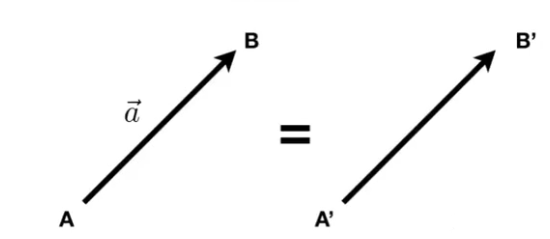2.1 向量的基本性质

• 写作 a ⃗ \vec a 或粗体的 a
• 由起点和终点表示 A B → \overrightarrow{AB}
• 具有方向和长度
• 没有绝对的开始位置

2.2 向量标准化

• 向量的长度写作 || a ⃗ \vec a ||

2.2.1 单位向量

• 单位向量是长度为1的向量
• 单位向量的获得： a ^ \hat a = a ⃗ \vec a /|| a → \overrightarrow a ||
• 使用单位向量来代表方向

2.3 向量的基本操作

2.1 向量相加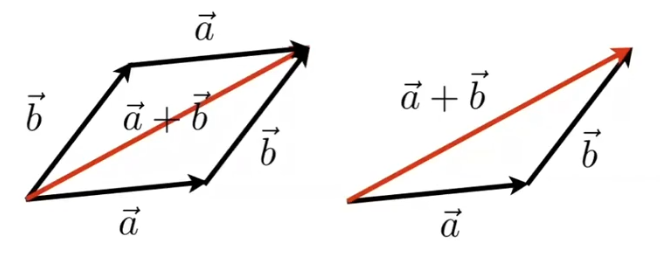• 几何上：平行四边形法则（左）和三角形法则（右）。
• 代数上：简单的坐标相加

2.2 向量的表示• 笛卡尔坐标系中的X和Y可以是任何向量（通常是垂直的）
• 一般向量表示是列向量
A = ( x y ) A= \begin{pmatrix} x \\ y \\ \end{pmatrix}
也可以转置来表示成行向量：
A T = ( x , y ) A^T=(x,y)
计算向量长度：
∥ A ∥ = x 2 + y 2 \Vert A \Vert=\sqrt {x^2+y^2}

2.3 向量的点乘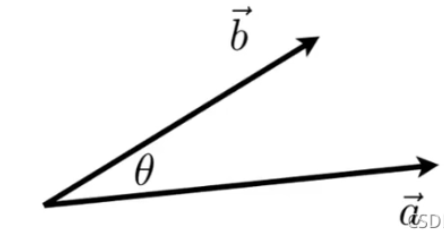2.3.1 点乘的基本运算

a → ⋅ b → = ∥ a → ∥ ∥ b → ∥ cos ⁡ θ \overrightarrow a · \overrightarrow b = \Vert \overrightarrow a \Vert \Vert \overrightarrow b \Vert \cos\theta

• 点乘可以获得夹角：
cos ⁡ θ = a → ⋅ b → ∥ a → ∥ ⋅ ∥ b → ∥ \cos\theta = \cfrac{\overrightarrow a · \overrightarrow b }{\Vert\overrightarrow a\Vert ·\Vert \overrightarrow b\Vert}
• 对于单位向量：
cos ⁡ θ = a ^ ⋅ b ^ \cos\theta = \hat a · \hat b
注意：向量点乘最后得到的是一个数。
2.3.2 点乘的性质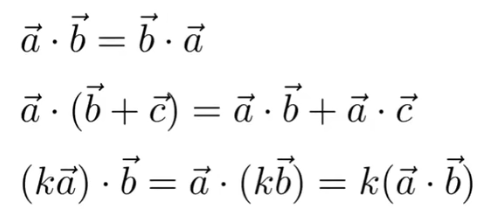2.3.3 在笛卡尔坐标系下的点乘运算：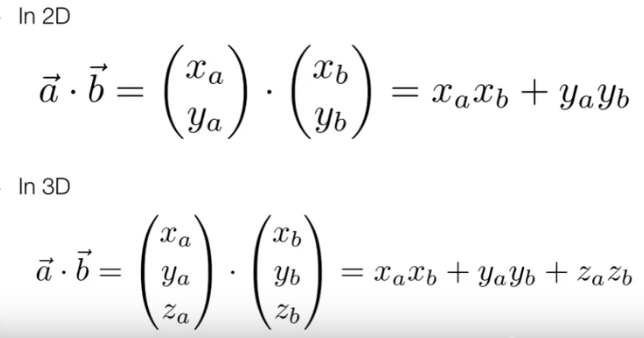2.3.4 点乘在图形学的应用
1. 找到两个向量的夹角：光来的方向、物体表面的法线、相机看的方向等等的夹角可以通过这个运算得到。
2. 找到一个向量投影到一个向量的结果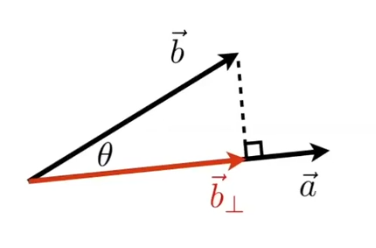其中， b → ⊥ \overrightarrow b_\bot b → \overrightarrow b a → \overrightarrow a 上的投影，且 b → ⊥ \overrightarrow b_\bot 是沿着 a → \overrightarrow a 或者 a ^ \hat a 的。有以下公式，其中k是向量长度：
b → ⊥ = k a ^ \overrightarrow b_\bot = k \hat a
k = ∥ b → ⊥ ∥ = k a ^ = ∥ b → ∥ cos ⁡ θ k = \Vert \overrightarrow b_\bot \Vert = k \hat a =\Vert \overrightarrow b \Vert\cos\theta
2.3.5 投影的用处：
1. 投影可以让一个向量分解成两个向量。（这两个向量互相垂直）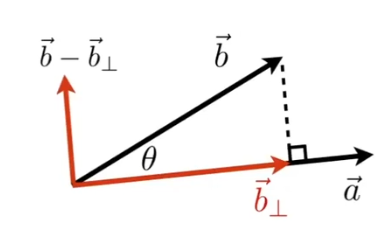如图所示，计算出投影后，垂直于向量 b ⃗ \vec b 投影方向的分量可以通过 b ⃗ − b ⃗ ⊥ \vec b - \vec b_\bot 获得。
2. 根据点乘的结果计算两个方向有多么接近
3. 判断前与后的信息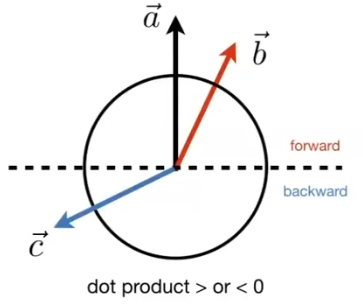如图所示，虚心将圆分割成两半，如果在forward区域内（点乘>0），就说 a ⃗ \vec a b ⃗ \vec b 是同向的，点乘为1说明完全同向，在backward区域内（点乘>0），就说 a ⃗ \vec a b ⃗ \vec b 是反向的，点乘为-1说明完全相反。点乘为0说明垂直。

2.4 向量的叉乘

2.4.1 基本概念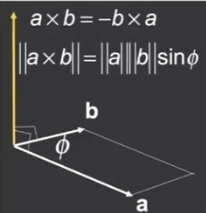1. 叉积是两个向量的垂直向量，即叉积得到的向量与另外两个向量都垂直。
2. 方向通过右手（螺旋）定则判断。能看出来向量的叉积不满足交换律，交换后的结果是相反的。
eg：判断从 a ⃗ \vec a b ⃗ \vec b 就让四指的方向从 a ⃗ \vec a b ⃗ \vec b ，大拇指的方向就是叉积的方向。
3. 对构建坐标系是有用的。如果三轴满足 x ⃗ × y ⃗ = z ⃗ \vec x \times \vec y = \vec z ，则这个坐标系是右手坐标系。，但是一些软件是左手坐标系，需要注意。
2.4.2 叉积的性质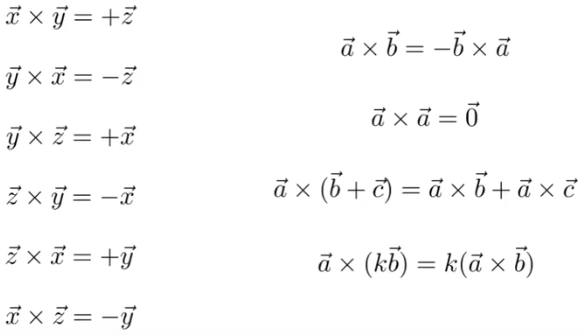2.4.3 笛卡尔坐标系下的叉乘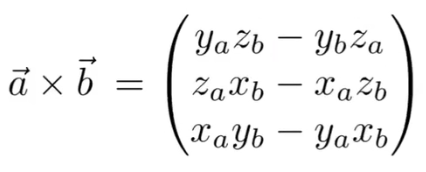叉乘也可以表示为矩阵形式：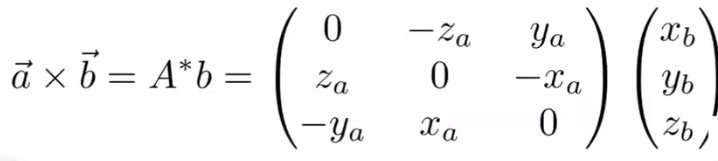2.4.3 叉乘在图形学中的作用
1. 判断左和右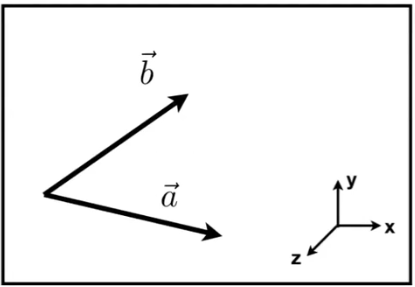如果 a ⃗ \vec a 顺时针旋转能到 b ⃗ \vec b ，就说 a ⃗ \vec a b ⃗ \vec b 的左侧，逆时针就是右侧。
计算 a ⃗ \vec a b ⃗ \vec b 的叉积，如果叉积的z是正的，就说明 b ⃗ \vec b a ⃗ \vec a 的左侧，叉积的z是负的，就是在右侧。
例如上图中， b ⃗ \vec b a ⃗ \vec a 的左侧，
2. 判断内与外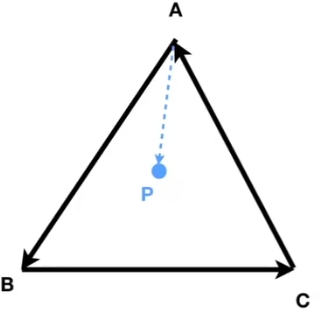如图所示， A B ⃗ × A P ⃗ > 0 \vec {AB} \times \vec {AP} > 0 ，说明P在AB的左边， B C ⃗ × B P ⃗ > 0 \vec {BC} \times \vec {BP} > 0 ，说明P在BC的左边 ， C A ⃗ × C P ⃗ > 0 \vec {CA} \times \vec {CP} > 0 ，说明P在CA的左边。

如果进行三个叉积运算的结果都为正或都为负，表明点都在三角形边的左边或者都在右边，故说明P在三角形内部。
否则说明点在三角形外面。

1. 直角坐标系的确定与坐标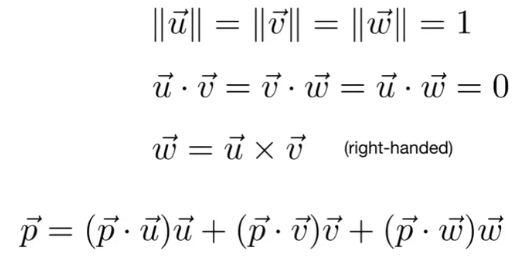3.矩阵

3.1 什么是矩阵

• 矩阵是数字的排列。（ m × n m \times n 的矩阵是m行，n列的。）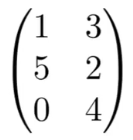• 矩阵的加法和数乘就是对矩阵的每一个元素都相加或都乘以这个数。

3.2 矩阵的乘积

3.2.1 可以做乘积的条件

第一个矩阵的列数必须等于第二个矩阵的行数，M行N列和N行P列做叉积，结果是M行P列的。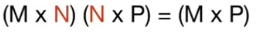3.2.2 乘积的运算

结果矩阵中（i，j）位置的数是A中第i行和B中第j列的点积。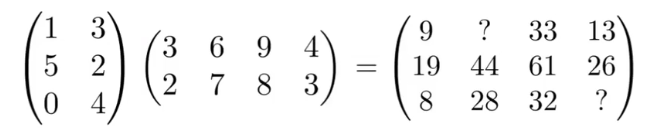eg：在上图中，26（结果矩阵2行4列）=5（左边2行）·4（右边4列）+2（左边2行）·3（右边4列）

3.2.3 矩阵乘积的性质

1. 没有交换律
2. 满足结合律（括号随便放，左右不能换）和分配律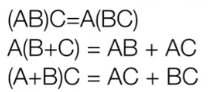3. 与向量做乘积时，把向量当作列向量（m × \times 1）
4. 矩阵的乘积对变化很重要
eg：求镜像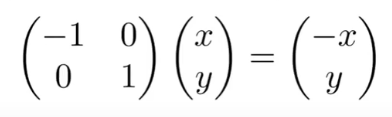3.3 矩阵的转置

• 矩阵的转置：交换矩阵的行和列（ij->ji）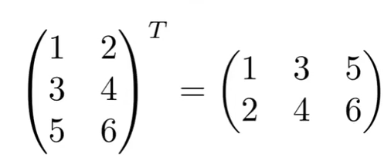• 转置的性质：
( A B ) T = B T A T (AB)^T = B^TA^T

3.4 特殊矩阵

3.4.1 单位矩阵

只有主对角线的元素不为0，且全都为1的矩阵，记为I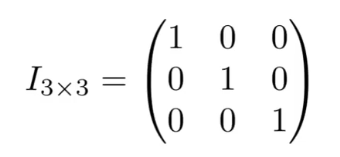3.4.2 矩阵的逆

• 逆矩阵的定义：与该矩阵相乘能得到单位矩阵的矩阵称为逆矩阵（两矩阵互逆）。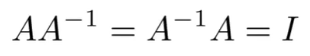• 逆矩阵的性质
( A B ) − 1 = B − 1 A − 1 (AB)^{-1} = B^{-1}A^{-1}

4. 向量点乘和叉乘的矩阵形式

4.1 点乘的矩阵形式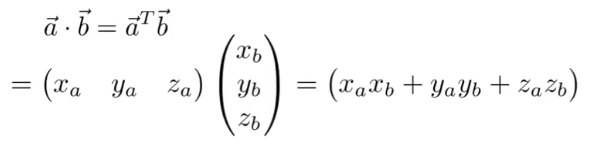4.2 叉乘的矩阵形式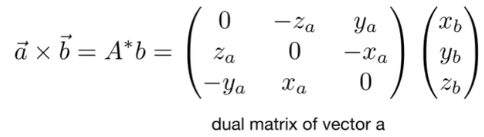其中 A ∗ A^* 是伴随矩阵。

展开全文线性代数
• import numpy as np #创建矩阵 a=np.array([[-1,-2,-1,0], #用mat 也OK [-2,4,2,7], [4,-1,0,2], ...# #矩阵的 # B=a.I # print(B) # # #矩阵的转置 # b=a.T # print(b) # #矩阵的秩 # b=np...
import numpy as np
#创建矩阵
a=np.array([[-1,-2,-1,0],  #用mat 也OK
[-2,4,2,7],
[4,-1,0,2],
[1,3,8,3]])
# #矩阵的逆
# B=a.I
# print(B)
#
# #矩阵的转置
# b=a.T
# print(b)

# #矩阵的秩
# b=np.linalg.matrix_rank(a)
# print(b)

#矩阵的特征值、特征向量 e特征值，f特征向量
e,f=np.linalg.eig(a)
print("特征值",e)
print("特征向量",f)

# #矩阵的伴随矩阵
# a_bs=B*np.linalg.det(a)
# print(a_bs)

展开全文• 进入了向量空间才算开始了线性代数的大门。 一、转置矩阵Permutation 转置矩阵适用于执行行交换的。回忆一下我们上一节讲到的A=LUA=LUA=LU分解，一个矩阵LULULU的LLL的特征非常明显，它是进行的行变换的直观矩阵： A...

进入了向量空间才算开始了线性代数的大门。几个重要的概念：

• 列空间包含所有列向量的线性组合，也就是所有可能 A x Ax 的结果向量，记作 C ( A ) C(A)
• 当且仅当 b b A A 的列空间， A X = b AX=b 才有解

一个 m × n m\times n 的系数矩阵 A A 的列空间有可能属于和向量分量一样多的空间 R m R^m ，也有可能只是子空间。

一、转置矩阵Permutation

转置矩阵适用于执行行交换的。回忆一下我们上一节讲到的 A = L U A=LU 分解，一个矩阵 L U LU L L 的特征非常明显，它是进行的行变换的直观矩阵：
A = L U = [ 1 0 0 0 X 1 0 0 X X 1 0 X X X 1 ] [ 1 X X X 0 1 X X 0 0 1 X 0 0 0 1 ] A=LU=\begin{bmatrix} 1&0&0&0\\ X&1&0&0\\ X&X&1&0\\ X&X&X&1 \end{bmatrix}\begin{bmatrix} 1&X&X&X\\ 0&1&X&X\\ 0&0&1&X\\ 0&0&0&1 \end{bmatrix} 如果考虑行交换，那么一个可逆矩阵的 A A 更广泛的消元过程应该表示为： P A = L U PA=LU ，Permutation矩阵的性质：

• P P 一定可逆的
• 逆等于转置

性质1，因为转置矩阵就是单位矩阵通过不同组合的行交换组成的，当然也可以乘以一个相反的行交换换回单位矩阵。性质2，举个直观的例子，假设一个转置矩阵

P = [ 1 0 0 0 0 0 1 0 0 1 0 0 0 0 0 1 ] P T = [ 1 0 0 0 0 0 1 0 0 1 0 0 0 0 0 1 ] P=\begin{bmatrix} 1&0&0&0\\ 0&0&1&0\\ 0&1&0&0\\ 0&0&0&1\\ \end{bmatrix}\quad P^T=\begin{bmatrix} 1&0&0&0\\ 0&0&1&0\\ 0&1&0&0\\ 0&0&0&1\\ \end{bmatrix} 它是一个单位矩阵第二和第三行的交换。 P P T = I PP^T=I 必然成立！

转置矩阵的数学表达
( A T ) i j = A j i (A^T)_{ij}=A_{ji}
对称矩阵(Symmetrix)的定义
A T = A A^T=A 数字是最直观的，一个对称阵 A A 应该是：
A = [ 3 1 7 1 2 9 7 9 4 ] A=\begin{bmatrix}3&1&7\\1&2&9\\7&9&4\end{bmatrix} 一个对称矩阵具有转置不变性。我们可以如何获取一个转置矩阵？答案是 R R T RR^T !矩阵总是可以乘以其转置，举个例子：
[ 1 3 2 3 4 1 ] [ 1 2 4 3 3 1 ] = [ 10 11 7 11 13 11 7 11 17 ] \begin{bmatrix} 1&3\\ 2&3\\ 4&1 \end{bmatrix}\begin{bmatrix} 1&2&4\\ 3&3&1\\ \end{bmatrix}= \begin{bmatrix}10&11&7\\11&13&11\\7&11&17\end{bmatrix} 这个是显然的，因为 ( R R T ) T = ( R T ) T R T = R R T (RR^T)^T=(R^T)^TR^T=RR^T 。转置等于其本身，符合转置矩阵的定义。

二、向量空间和子空间

定义： R n R^n 空间是所有 n n 个分量列向量的组合 R 5 R^5 表示的是五个分量的列向量集合，如
[ 3 4 8 1 6 ] [ 3 4 − 1 3 2 ] \begin{bmatrix} 3\\4\\8\\1\\6 \end{bmatrix}\quad \begin{bmatrix}3\\4\\-1\\3\\2 \end{bmatrix}
如果你能枚举所有的这样的向量，那么这个集合组成的就是 R n R^n 空间。

2.1 向量空间

向量空间是向量集合，在向量集合满足一定规则的向量称为子空间。

子空间是一系列满足一定运算规则构成的所有向量集，这个规则是满足以下条件，

• 任取向量空间中的两个向量 v v w w v + w v+w 仍然在这个空间;
• 任取一个标量 c c c v cv 属于这个空间；

换句话说，所有向量线性组合都是在子空间。

R 2 R^2 就是一个空间向量，其向量集合是所有二维实向量，对于其中的如：
[ 3 2 ] [ 0 0 ] [ π e ] \begin{bmatrix}3\\2\end{bmatrix}\quad \begin{bmatrix}0\\0\end{bmatrix}\quad \begin{bmatrix}\pi\\e\end{bmatrix} 无论我们这些二维实向量做何线性运算，其结果仍然在空间 R 2 R^2 内。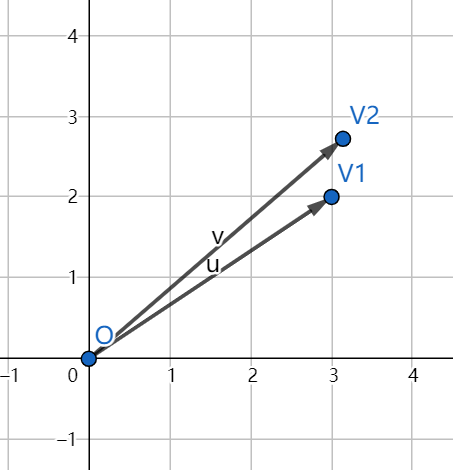R 2 R^2 空间就是平面空间，随意选取平面上向量集合，如截取 R 2 R^2 平面一部分：第一象限，这个平面是否是空间向量呢？不是的，画个图看看：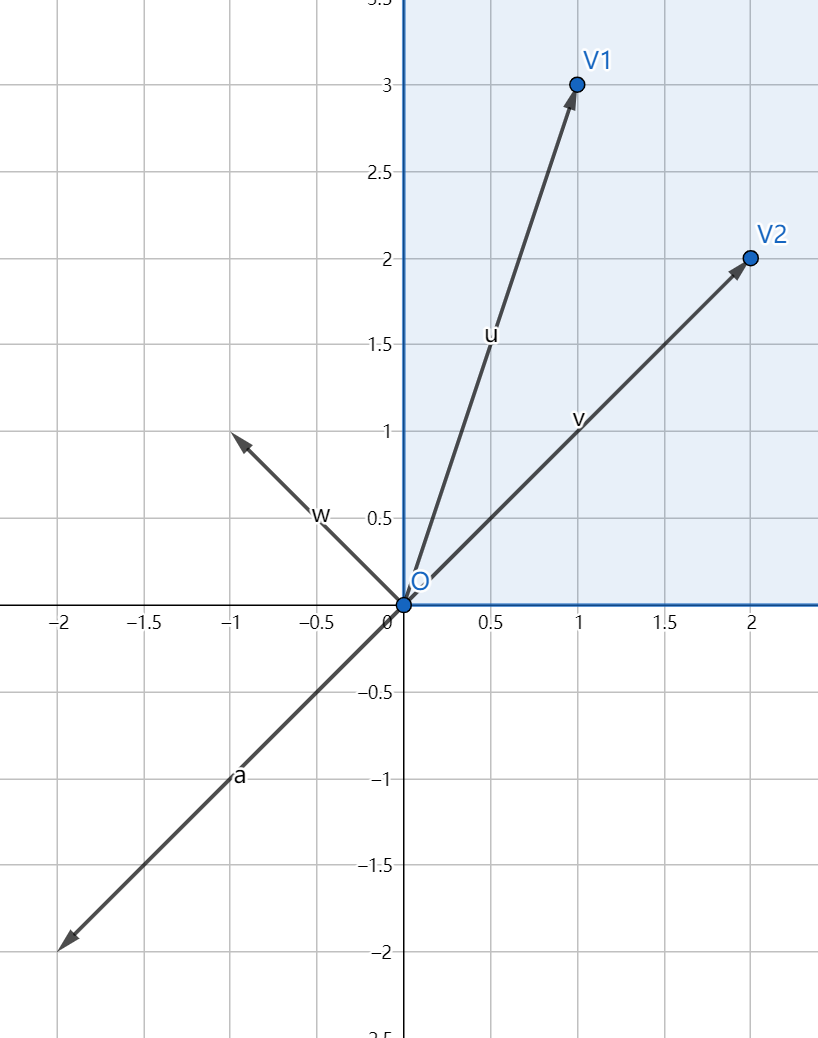w = u − v w=u-v 显然已经不在指定的第一象限，不符合向量空间的定义。

2.2 子空间

前面在 R 2 R^2 空间划取第一象限不是一个向量空间。那么在一个向量空间中任意划取一部分，是否有可能组成一个向量空间？答案是肯定的！！如一个过原点的直线空间！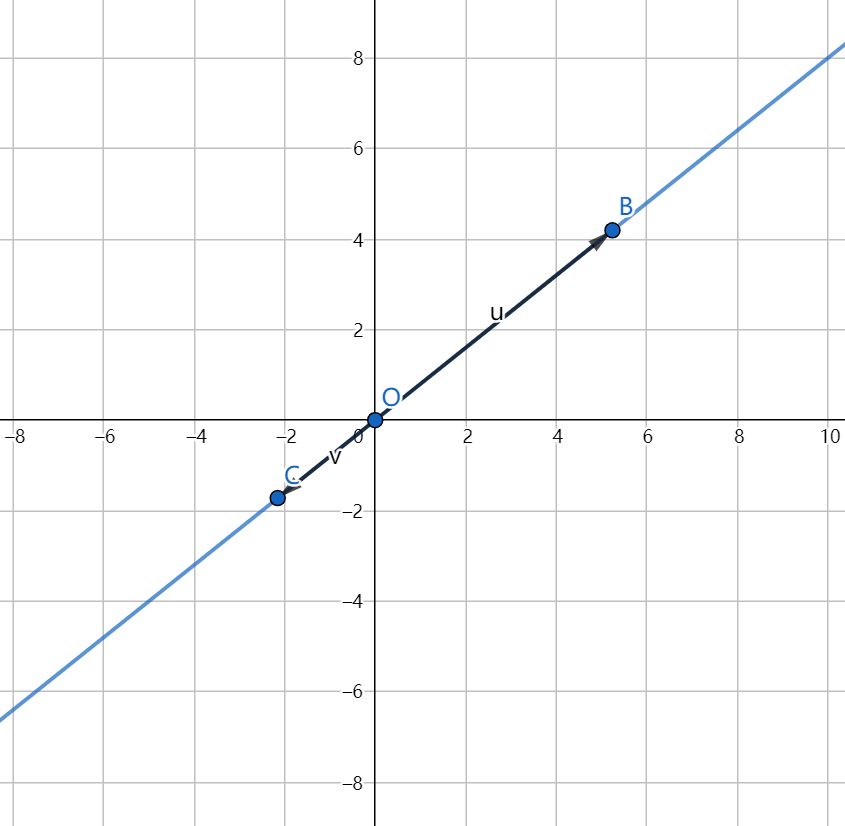我们随便在蓝色的空间任意选取一个点组成向量，无论我们如何线性组合它仍然属于这个蓝色区域，符合向量空间的定义！能够再举一个例子吗？当然可以，如零向量空间，不过逆只能取到一个向量，零向量的线性组合仍然是零向量。ok！至此，我们找到了 R 2 R^2 所有的三种子空间（线面）都被我们找到了！

对于 R 3 R^3 也是一个向量空间，三个实数构成一个向量，向量间的线性运算并不会离开 R 3 R^3 空间，如：
[ 3 2 1 ] [ 0 0 0 ] [ π e 4 ] \begin{bmatrix}3\\2\\1\end{bmatrix}\quad \begin{bmatrix}0\\0\\0\end{bmatrix}\quad \begin{bmatrix}\pi\\e\\4\end{bmatrix}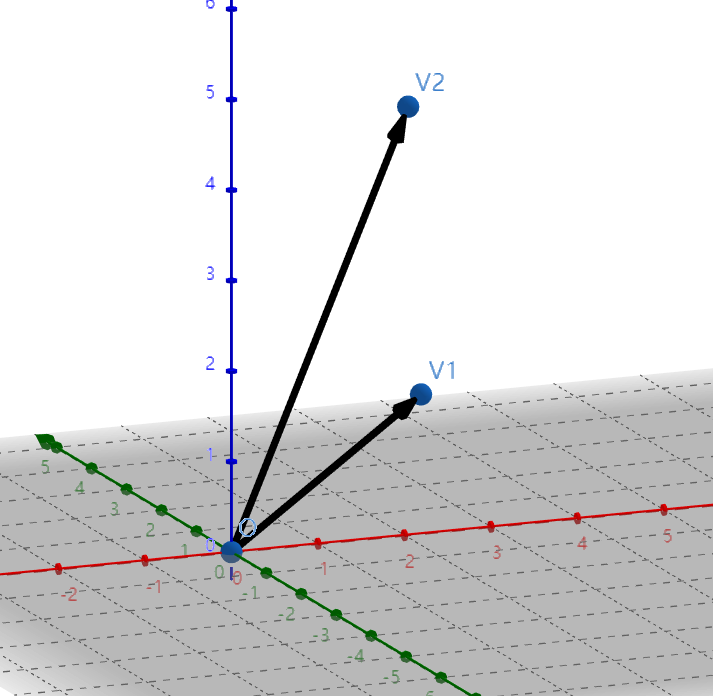同样的我们也对 R 3 R^3 的子空间，进行查找。第一个，过原点的平面：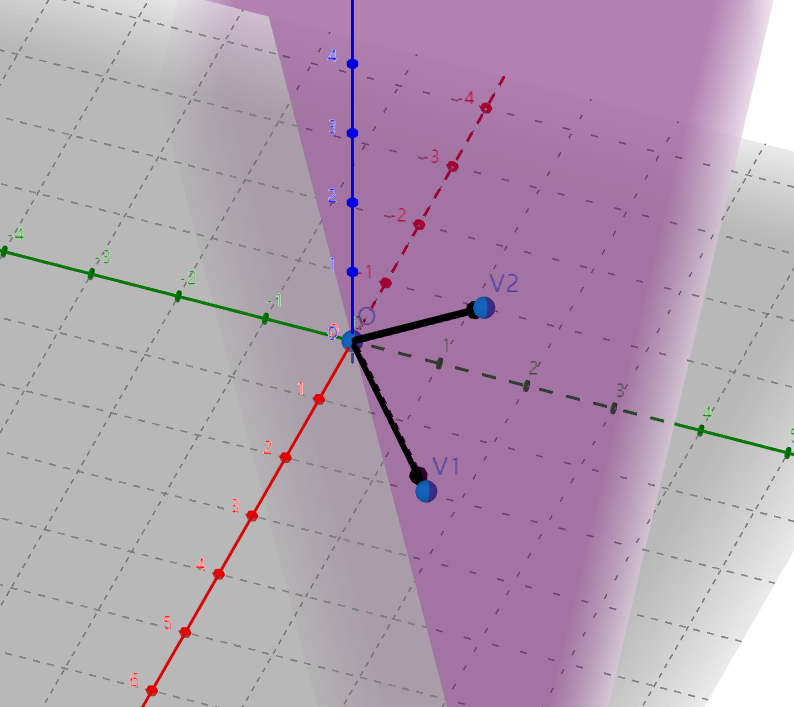在这个平面上任取向量进行线性组合，其结果仍然再这个平面上，是一个子空间。
过原点的直线：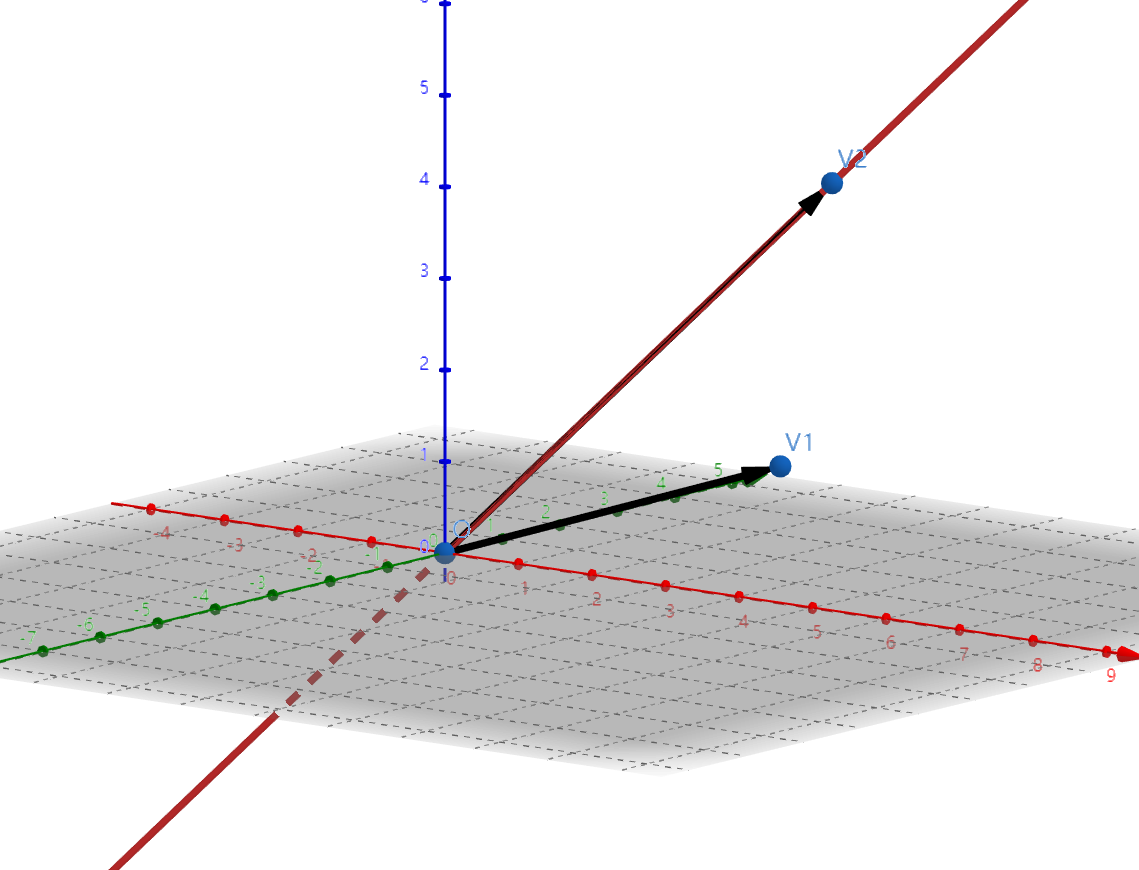显然，同理，它是一个子空间。最后，原点，也是一个子空间。

为什么 R 2 R^2 R 3 R^3 的子空间都包含零向量？对于更高维度的子空间是否也包含零向量？原因很简单，如果你是一个向量空间，任取一个向量 v v ，对于任意实数必须有： k v kv 成立，如果我们取 k = 0 k=0 ，他就是一个零向量！这也说明，如果一个空间连零向量都没有那他一定不是向量空间。

2.3 矩阵的列空间

矩阵的列向量的所有的线性组合必然在某个向量空间中，我们将列向量的线性组合的所有可能称为矩阵的列空间。举个例子:
[ 1 3 2 3 4 1 ] \begin{bmatrix} 1&3\\ 2&3\\ 4&1 \end{bmatrix} 这个矩阵构成的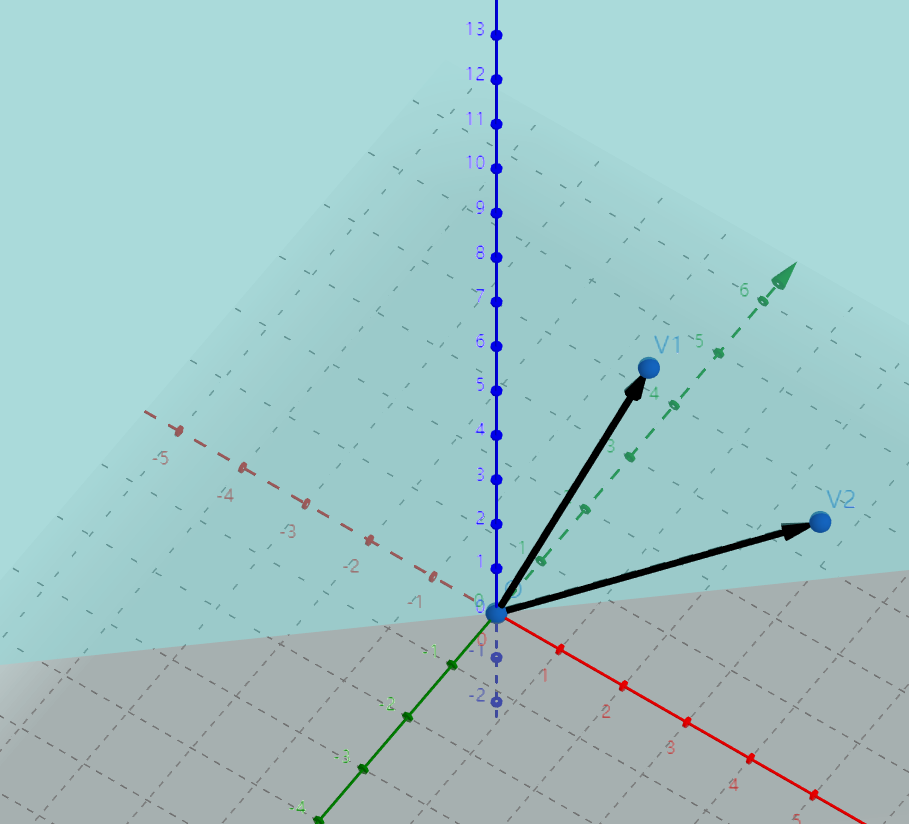矩阵的列空间是一个过原点的 R 3 R^3 子空间：平面。当然如果列向量是一个共线向量，那么子空间则是过原点的 R 3 R^3 子空间：直线。我们矩阵列构成的空间称为矩阵到列空间，记作 C ( A ) C(A)

展开全文线性代数
• 由于向量仅仅只有1行，那么通过一个变量i来指定获取第i行的数据，很容易理解。 矩阵运算 矩阵加法 矩阵的加法，要求两个矩阵或者是多个矩阵，要求所有的矩阵的列和行都是一样的，例如都是3X2的矩阵，或者是5x8...
• 文章目录矩阵转置np.transpose(a)矩阵求 S**-1奇异值分解 SVD余弦相似度 矩阵转置np.transpose(a) import numpy as np a= [0.26,0,0.52,0,0,0,0,0,0,0,0] np.transpose(a) np.shape(np.transpose(a)) 矩阵求 S*...python numpy
• 目录前言符号约定向量的基本性质 【定义，基向量，线性相关/无关*，向量点积】矩阵的基本性质 【转置，广播，线性变换】矩阵基本运算 【矩阵相乘，矩阵点积】行列式 【概念，性质，右手法则，行列式计算】矩阵 ...c++矩阵转置 ker矩阵是什么意思 两矩阵相乘的秩的性质
• 矩阵的逆和转置

千次阅读 2020-06-05 14:42:53
矩阵的 （inverse matrix） (AB)−1=B−1A−1(A B)^{-1}=B^{-1} A^{-1}(AB)−1=B−1A−1 (ABC)−1=C1B−1A−1(A B C)^{-1}=C^{1} B^{-1} A^{-1}(ABC)−1=C1B−1A−1 ...矩阵的转置 (A−1)T=(AT)−1\le..线性代数
• 若存在一个矩阵A-1使得AA-1 = E，则A-1为A矩阵 条件：矩阵非奇异=矩阵对应的行列式不为0=满秩=行列向量线性无关 矩阵的几种求法 待定系数法 待定系数法顾名思义是一种求未知数的方法。将一个多项式表示成另一种...
• diag(A) 提取矩阵A主对角线元素，产生一个列向量。 diag(A,k) 提取第k条对角线上的元素 k=0是中间的那条对角线 （2）构造对角矩阵 diag(V) 产生一个主对角线为V的对角阵 diag(V,k) 向量v为第...matlab
• 转置矩阵 在线性代数中，矩阵AAA的转置（transpose）是另一个矩阵ATA^{\mathrm {T} }AT（也写做AtrA^{tr}Atr, tAt_AtA​或A′A^′A′）由下列等价动作建立： 把AAA的横行写为ATA^{\mathrm {T} }AT的纵列 把AAA的纵列...线性代数 矩阵
•matlab
• 矩阵的转置、求、旋转、翻转 inv(A)：求矩阵A的矩阵； 转置：A.'为矩阵A的转置，A’为矩阵A的共轭转置； rot90(A,k)：将矩阵A时针方向旋转90°的k倍，k为1时可省略； fliplr(A)：将矩阵A左右翻转； flipld(A)...matlab